Example Questions

Example Question #678 : Sat Mathematics

How many times does the equation below cross the x-axis?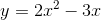Explanation:

You can solve this problem two ways.

3. You can solve for where the graph crosses the x-axis by setting the equation equal to zero, factoring, and solving.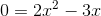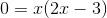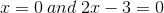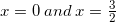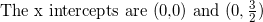2. You can quickly sketch the graph by choosing some x values and solving for y.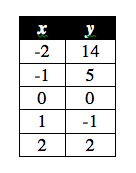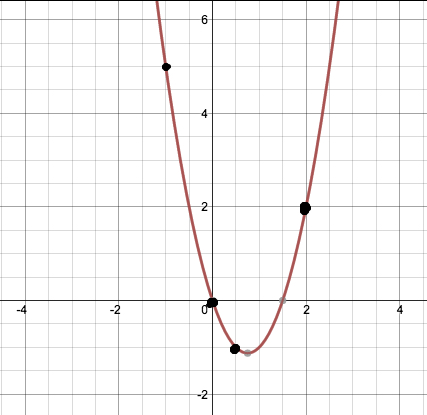We see that the graph passes the x-axis twice.

Example Question #679 : Sat Mathematics

Let f(x) = x2. By how many units must f(x) be shifted downward so that the distance between its x-intercepts becomes 8?

16

4

12

8

2

16

Explanation:

Because the graph of f(x) = x2 is symmetric about the y-axis, when we shift it downward, the points where it intersects the x-axis will be the same distance from the origin. In other words, we could say that one intercept will be (-a,0) and the other would be (a,0). The distance between these two points has to be 8, so that means that 2a = 8, and a = 4. This means that when f(x) is shifted downward, its new roots will be at (-4,0) and (4,0).

Let g(x) be the graph after f(x) has been shifted downward. We know that g(x) must have the roots (-4,0) and (4,0). We could thus write the equation of g(x) as (x-(-4))(x-4) = (x+4)(x-4) = x2 - 16.

We can now compare f(x) and g(x), and we see that g(x) could be obtained if f(x) were shifted down by 16 units; therefore, the answer is 16.

Example Question #680 : Sat Mathematics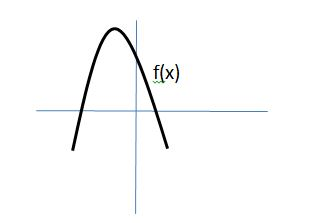The graph of f(x) is shown above. If f(x) = ax2 + bx + c, where a, b, and c are real numbers, then which of the following must be true:

I. a < 0

II. c < 0

III. b2 – 4ac < 0

I only

I and III only

II and III only

II only

I and II only

I only

Explanation:

Let's examine I, II, and III separately.

Because the parabola points downward, the value of a must be less than zero. Thus, a < 0 must be true.

Next, let's examine whether or not c < 0. The value of c is related to the y-intercept of f(x). If we let x = 0, then f(x) = f(0) = a(0) + b(0) + c = c. Thus, c is the value of the y-intercept of f(x). As we can see from the graph of f(x), the y-intercept is greater than 0. Therefore, c > 0. It is not possible for c < 0. This means choice II is incorrect.

Lastly, we need to examine b2 – 4ac, which is known as the discriminant of a quadratic equation. According to the quadratic formula, the roots of a quadratic equation are equal to the following:

Notice, that in order for the values of x to be real, the value of b2 – 4ac, which is under the square-root sign, must be greater than or equal to zero. If b2 – 4ac is negative, then we are forced to take the square root of a negative number, which produces an imaginary (nonreal) result. Thus, it cannot be true that b2 – 4ac < 0, and choice III cannot be correct.

Only choice I is correct.

Example Question #1 : Transformation

Let f(x) = -2x2 + 3x - 5. If g(x) represents f(x) after it has been shifted to the left by three units, and then shifted down by four, which of the following is equal to g(x)?

-2x^2 - 9
-2x^2 + 3x - 12
-2x^2 + 15x - 36
-2x^2 + 31x - 124
-2x^2 - 9x - 18
Correct answer: -2x^2 - 9x - 18
Explanation:

We are told that g(x) is found by taking f(x) and shifting it to the left by three and then down by four. This means that we can represent g(x) as follows:

g(x) = f(x + 3) - 4

Remember that the function f(x + 3) represents f(x) after it has been shifted to the LEFT by three, whereas f(x - 3) represents f(x) after being shifted to the RIGHT by three.

f(x) = -2x2 + 3x - 5

g(x) =  f(x + 3) - 4 = [-2(x+3)2 + 3(x+3) - 5] - 4

g(x) = -2(x2 + 6x + 9) + 3x + 9 - 5 - 4

g(x) = -2x2 -12x -18 + 3x + 9 - 5 - 4

g(x) = -2x- 9x - 18 + 9 - 5 - 4

g(x) = -2x- 9x - 18

The answer is -2x- 9x - 18.

Example Question #2 : Transformation

Let f(x) be a function. Which of the following represents f(x) after it has been reflected across the x-axis, then shifted to the left by four units, and then shifted down by five units?

–f(x – 4) – 5

–f(x – 5) – 4

f(x + 4) – 5

–f(x + 5) – 4

–f(x + 4) – 5

–f(x + 4) – 5

Explanation:

f(x) undergoes a series of three transformations. The first transformation is the reflection of f(x) across the x-axis. This kind of transformation takes all of the negative values and makes them positive, and all of the positive values and makes them negative. This can be represented by multiplying f(x) by –1. Thus, –f(x) represents f(x) after it is reflected across the x-axis.

Next, the function is shifted to the left by four. In general, if g(x) is a function, then g(x – h) represents a shift by h units. If h is positive, then the shift is to the right, and if h is negative, then the shift is to the left. In order, to shift the function to the left by four, we would need to let h = –4. Thus, after –f(x) is shifted to the left by four, we can write this as –f(x – (–4)) = –f(x + 4).

The final transformation requires shifting the function down by 5. In general, if g(x) is a function, then g(x) + h represents a shift upward if h is positive and a shift downward if h is negative. Thus, a downward shift of 5 to the function –f(+ 4) would be represented as –f(+ 4) – 5.

The three transformations of f(x) can be represented as –f(+ 4) – 5.

The answer is –f(+ 4) – 5.

Example Question #3 : Transformation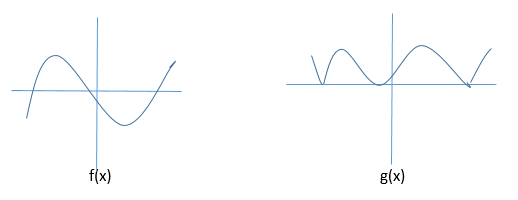The graphs of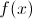and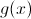are shown above. Which equation best describes the relationship betweenand?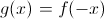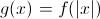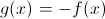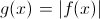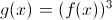Explanation: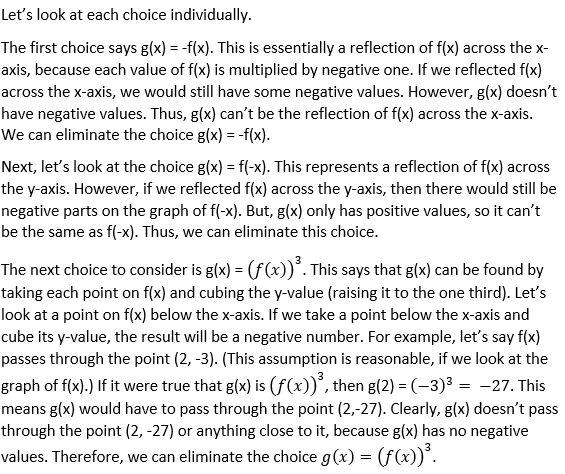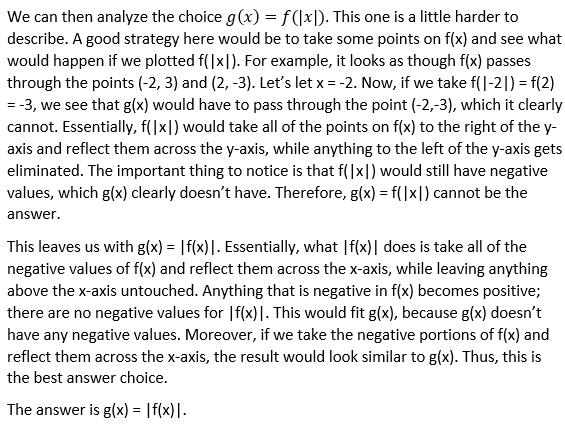Example Question #4 : Transformation

If the point (6, 7) is reflected over the line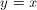and then over the x-axis, what is the resulting coordinate?

(7, 6)

(–6, –7)

(6, 7)

(6, –7)

(7, –6)

(7, –6)

Explanation:

A reflection over the lineinvolves a switching of the coordinates to get us (7, 6). A reflection over the x-axis involves a negation of the y-coordinate. Thus the resulting point is (7, –6).

Example Question #5 : Transformation

You are looking at a map of your town and your house is located at the coordinate (0,0). Your school is located at the point (3,4). If each coordinate distance is 1.3 miles, how far away is your school?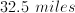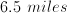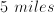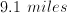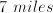Explanation:

The coordinate length between you and your school is equivalent to the hypotenuse of a right triangle with sides of 3 and 4 units: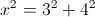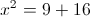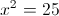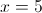The distance is 5 coordinate lengths, and each coordinate length corresponds to 1.3 miles of distance, so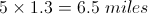Example Question #6 : Transformation

Line m passes through the points (–4, 3) and (2, –6). If line q is generated by reflecting m across the line y = x, then which of the  following represents the equation of q?

2x + 3y = 6

3x + 2y = 6

3x + 2y = 18

2x + 3y = –6

–2x + 3y = 6

2x + 3y = –6

Explanation:

When a point is reflected across the line y = x, the x and y coordinates are switched. In other words, the point (a, b) reflected across the line y = x would be (b, a).

Thus, if line m is reflected across the line y = x, the points that it passes through will be reflected across the line y = x. As a result, since m passes through (–4, 3) and (2, –6), when m is reflected across y = x, the points it will pass through become (3, –4) and (–6, 2).

Because line q is a reflection of line m across y = x, q must pass through the points (3, –4) and (–6, 2). We know two points on q, so if we determine the slope of q, we can then use the point-slope formula to find the equation of q.

First, let's find the slope between (3, –4) and (–6, 2) using the formula for slope between the points (x1, y1) and (x2, y2).slope = (2 – (–4))/(–6 –3)

= 6/–9 = –2/3

Next, we can use the point-slope formula to find the equation for q.

y – y1 = slope(x – x1)

y – 2 = (–2/3)(x – (–6))

Multiply both sides by 3.

3(y – 2) = –2(x + 6)

3y – 6 = –2x – 12

2x + 3y – 6 = –12

2x + 3y = –6

The answer is 2x + 3y = –6.

Example Question #7 : Transformation

Solve each problem and decide which is the best of the choices given.

What is the amplitude of the following function?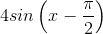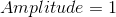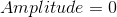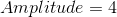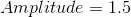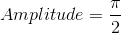Explanation:

The amplitude is always the number in front of the trig function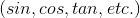.

The general equation to remember is,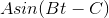whereis amplitudeis period of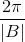andis the phase shift.

In this case it's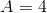.